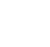# Computing for profits

There are three methods of calculating the soundness of your investment decision.
By Bay Cabrera |

Profit is a major goal of most businesses big and small. If you organize a business of your own, you do so in the hope it will operate at a profit. A profitable operation is essential if your business is to survive and succeed, hence you must think long and hard before making any investment decisions. After all, you invest money mainly to make money out of the investment.

The three elements necessary to judge the attractiveness of any investment are the investment itself, the operating cash flows, and the economic life of the investment project.

The investment refers to the cash outlay or the amount of owner’s capital to be put into the project. The operating cash flows are the net economic benefits or after-tax profits resulting from the investment. The economic life of an investment is the time the investor must wait before reaping the benefits of his investment.

The three common methods of evaluating investment decisions are the payback period, net present value, and internal rate of return.

PAYBACK PERIOD

The payback period is the time required for the cash flow or proceeds produced by the investment to equal the original cash outlay that the investment requires. If an investment is expected to produce a cash flow that is constant from year to year, you may determine the payback period by dividing the original cash outlay by the amount of the annual cash proceeds expected.

If the cash flow is not equal from year to year, you determine the payback period by adding the expected proceeds in each succeeding year until the total is equal to the original outlay. The investment project is acceptable only if the payback period is less than the specified cut-off period. The income beyond the payback date is ignored; those cash flows before the payback date are given equal weight—meaning the cash flow in year one is treated the same way as the cash flow received in year 20.

The advantage of this method is that it is simple to calculate and it provides an insight on risk. Its disadvantage is that it fails to consider the cash proceeds after the payback period, it does not consider the differences in the timing of cash flows before the payback period, and it is biased against investment projects that do not yield their highest returns in the early years.

NET PRESENT VALUE

Net present value is the present value of all future net cash flows discounted at the cost of capital or minimum required rate of return—less the cost of the initial investment. Sometimes, net present value is referred to as discounted cash flow analysis.

The net present value is calculated using the following steps:

• Choose the appropriate interest rate as the cost of capital for your business. You may use current bond yields or market interest rates to choose your rate.

• Compute the present value of the future cash flows (both in and out) produced by the investment by multiplying the future cash flows per period by the corresponding present value factor for the period. The formula for calculating the present value factor of an amount to be received n years hence at an interest rate i is:

1

(1+i)n

You may also use the present value table—it contains the present value factors for various interest rates per year—for this purpose.

• Subtract the cost of the investment in period zero from the present value of inflows and outflows (net cash inflows). The result is the net present value of the investment.

## Latest Articles

Close